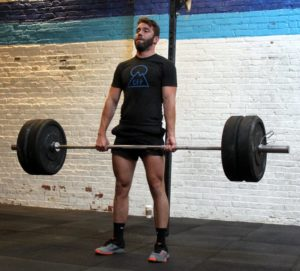## Thursday, November 29, 2018

### Thursday, November 29, 2018as many rounds as possible in 16 minutes:
400m run
10x unbroken back squat @ 205/135-lbs.

### 1 Comment

1.–Scores–
Matthew W.: 6 rounds (155-lbs.; sub: from rack)
Anna G.: 4 rounds + 150m (75-lbs.)
Kate H.: 4 rounds + 3x squat (75-lbs.; sub: row)
Al C.: 4 rounds (155/135-lbs.)
Hari P.: 5 rounds + 100m (155-lbs.)
Borja G.: 5 rounds + 50m (35-lb. dbs; sub: 16x weighted step-up, 18″)
Charles B.: 3 rounds + 9x squat (155-lbs.; sub: from rack)
Collin S.: 4 rounds + 150m (105-lbs.)
Matt H.: 4 rounds + 300m (115-lbs.)
Kevin K.: 4 rounds + 150m (115-lbs.)
Chase H.: 5 rounds + 400m (135-lbs.)
Sean Q.: 4 rounds + 6x squat (155-lbs.; sub: row)
Aaron B.: 4 rounds + 4x squat (115-lbs.)
Miranda B.: 4 rounds + 70m (85-lbs.; sub: row, from rack)
Sara R.: 7 rounds + 250m (45-lbs.; sub: 200m row/200m run, prom front squat)
Sean F.: 5 rounds (135-lbs.)
Will H.: 4 rounds + 300m (95-lbs.)
David C.: 4 rounds + 400m (115-lbs.; sub: from rack)
Thi N.: 3 rounds + 50m (115-lbs.)
Adrian P.: 4 rounds + 75m (115-lbs.)
Ryan A.: 4 rounds + 400m (105-lbs.)
Drew P.: 5 rounds (115-lbs.)
Andrew B.: 4 rounds + 375m (75-lbs.)
Elise C.: 4 rounds + 125m (115-lbs.)
Elena P.: 3 rounds + 3x squat (40-lbs.)
Alissa G.: 10′ EMOM x 5 back squat (125-lbs.; sub: from rack)
RJ A.: 4 rounds + 5x back squat (95-lbs.)
Charlie S.: 5 rounds + 250m (95-lbs.)
Andrew Z.: 4 rounds + 300m (155-lbs.)
Will O.: 5 rounds + 200m (105-lbs.)
Cristian D.: 4 rounds + 200m (85-lbs.)
Buffy M.: 5 rounds (95-lbs.; sub: row, deadlift)
Patrick J.: 4 rounds + 350m (125-lbs.; sub: front squat)
Melody F.: 4 rounds + 50m
Patrick M.: 4 rounds + 100m (115-lbs.; sub: front squat)
Marcus B.: 4 rounds + 400m (155-lbs.)
Josh M.: 4 rounds + 4x back squat (115-lbs.; sub: from rack, prom)
Chris H.: 4 rounds + 200m
John La.: 4 rounds + 1 squat (105-lbs.)
Dan A.: 3 rounds + 2′ bike (35-lbs.; sub: 1/2 run + 1/2 2′ bike)
Joya TD.: 4 rounds + 5 squat (75-lbs.)
Anna Go.: 3 rounds + 3 squat (75-lbs.)
Rebekka E.: 4 rounds + 150m run (95-lbs.; sub: from rack)
Francisco L.: 4 rounds + 250m run (135-lbs.)
Michael H.: 4 rounds + 400m (155-lbs.)
Charlie K.: 4 rounds + 200m run (95-lbs.)
Mark Co.: 4 rounds (165-lbs.)
Joe T.: 5 rounds + 175m run (175-lbs.)
Amy S.: 4 rounds + 300m run (135-lbs.)
Christian M.: 4 rounds + 250m run (85-lbs.; sub: lunges)
Matt K.: 3 rounds + 2 squats (135-lbs.; sub: from rack)
Lily S.: 4 rounds + 100m run (55-lbs.)
Kat S.: 4 rounds + 125m run (sub: 15x air squat + 1-min. plank)
Daniel D.: 4 rounds + 260m run (105-lbs.)
Katie G.: 3 rounds + 200m run (55-lbs.)